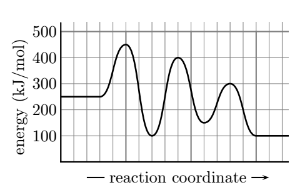# Problem: The potential energy diagram shown to the right is for a specific reaction scheme. How many steps are in the reaction mechanism and which step is the limiting step? A. 2 steps , 2nd step limitingB. 3 steps , 1st step limitingC. 2 steps , 1st step limitingD. 3 steps , 2nd step limitingE. 3 steps , 3rd step limitingF. 1 step , 1st step limiting

###### FREE Expert Solution

We’re being asked to identify how many steps are in the reaction mechanism and which step is the limiting step

Recall that an energy diagram is usually read from left to right

The components of an energy diagram are:

• Reactants: are placed on the left/beginning of the energy diagram

• Products: are placed on the right/end of the energy diagram

• Rate-limiting transition state: is the transition state with the highest energy in the energy diagram

• Non-limiting transition state: is the transition state with the lower energy in the energy diagram

• Intermediates: are formed in between steps in the energy diagram

• Energy change (ΔH or ΔG˚): is the difference in energy between products and reactants

• Activation energy (Ea): is the difference in energy between reactants and transition state

82% (353 ratings)###### Problem Details

The potential energy diagram shown to the right is for a specific reaction scheme. How many steps are in the reaction mechanism and which step is the limiting step?

A. 2 steps , 2nd step limiting

B. 3 steps , 1st step limiting

C. 2 steps , 1st step limiting

D. 3 steps , 2nd step limiting

E. 3 steps , 3rd step limiting

F. 1 step , 1st step limiting